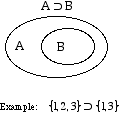index: click on a letter A B C D E F G H I J K L M N O P Q R S T U V W X Y Z A to Z index index: subject areas numbers & symbols sets, logic, proofs geometry algebra trigonometry advanced algebra & pre-calculus calculus advanced topics probability & statistics real world applications multimedia entrieswww.mathwords.com about mathwords website feedback

 Superset Set C is a superset of set D if set C contains all of the elements (if any) of set D. This is written C ⊃ D. Note: Every set is a superset of the empty set.See also